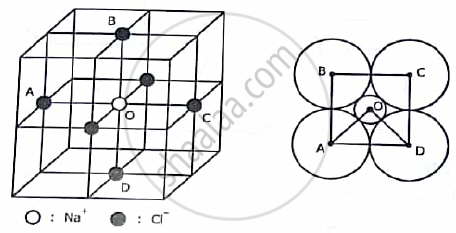# Calculate the critical radius ratio of an ionic crystal in ligancy-6 . what is the maximum size of cation in ligancy 6 configuration when size of anion is 2.02A°? - Applied Physics 1

Short Note

Calculate the critical radius ratio of an ionic crystal in ligancy-6 . what is the maximum size of cation in ligancy 6 configuration when size of anion is 2.02A°?

#### Solution

OCTAHEDRAL CONFIGURATION:

The octahedral configuration of neighbouring anions is found in NaCl structure. Here four anions A, B ,C and D are arranged at the corners of a square with the cation O at the centre of the square. Two more anions are situated in front and at the back of the cation. The centres of all six anions form an octahedron.

Here in ΔBOC,<BOC= 90°,BC=2rA ,OB= rC+ rA and<BCO=45°,

Hence, = (BO )/( BC )cos45°

Or  (r_c+r_A)/(2r_A) = 1/sqrt(2)The critical radius ratio here is,

 r_c/r_A = 0.414

NUMERICAL:

Given data  :- r A(max) = 2.02A° ,ligancy=6.

Formula :- for ligancy 6, (("^rc) /r_A)=0.732

Calculations : - r_c= r_A ×0.732 = 2.02 × 0.732 = 1.478A°
Answer :-r_C=1.478A°

Concept: Study of characteristics of unit cell of Diamond, ZnS, NaCl and HCP;
Is there an error in this question or solution?
2016-2017 (December) CBCGS

Share